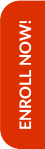Olympiads are the stepping stones to achieve better results in the competitive world that lies ahead in the life of the child. Math Olympiad examinations help students to improve their mathematical skills along with their analytical and problem solving abilities.

Hence, Olympiad Success Live has designed the course for Math Olympiad for class 8 in such a way that the foundation of the child is built up. For this, we have done great efforts in finding the tutor for class 8 Math Olympiad with relevant background and experience.

If you are interested in purchasing this course, then please Enrol Now. You will be redirected to the batch detail page, wherein you can see all the details like batch start and demo dates, fess and the registration link related to Math Olympiad for class 8 course.

##### Syllabus

Rational Numbers

• Rational number and its examples
• Natural number, Whole number and integer
• Properties of rational numbers (Using identities)
• Representation of rational number on the number line

Linear Equation in One Variable

• Equation, linear equations
• Linear equation in one variable
• Solution of a linear equation
• Laws of equality
• Problems based on linear equations

• Classification of polygons
• Convex and concave polygon
• Regular and irregular polygon
• Angle sum property
• Interior and exterior angles of a regular polygon
• Constructions of triangles and quadrilaterals

Squares and Square Root, Cube and Cube Root

• Square and square root
• Square, perfect square and square root
• Properties of squares
• Estimating the squares of numbers
• Determining the square and square roots of positive numbers
• Cube and cube root
• Cube, perfect cube and cube roots
• Estimating the cubes of numbers
• Determining the cube and cube roots of positive numbers
• Estimating the cubes of numbers

Comparing Quantities

• Ratio and proportion
• Terms of a ratio
• Ratio in simplest form
• Comparison of ratios
• Continued proportion
• Problems on property - product of extremes = product of means
• Problems on percentage
• Profit and loss
• Simple interest and compound interest

Algebraic Expressions and Identities

• Algebraic expression
• Definition of algebraic expression
• Like and unlike terms
• Polynomial
• Degree of a polynomial
• Types of polynomials
• Multiplication of polynomials
• Important identities- (a + b)2 = a2 + 2ab + b2
• (a – b)2 = a2 - 2ab + b2
• (a + b) (a – b) = a2 – b2

Mensuration

• Area and Perimeter
• Definition of area and perimeter
• Area and perimeter of rectangle
• Area and perimeter of square
• Area of four walls of room
• Area and perimeter of triangle
• Area and perimeter of parallelogram
• Area and circumference of circle
• Area of sector and segment
• Length of arc
• Volume
• Volume of cube, cuboid and cylinder
• Total surface area of cube, cuboid and cylinder
• Lateral surface area of cube, cuboid and cylinder
• Diagonal of cube and cuboid

Exponents and Powers

• Exponential equation
• Standard form of numbers
• Laws of exponents (Integers and rational numbers)
• Positive and negative integral exponent of a rational number

Direct and Inverse Proportions

• Unitary method
• Direct proportion
• Inverse proportion

Factorization

• Definition of factorization
• Important identities- (a + b)2 = a2 + 2ab + b2
• (a – b)2 = a2 - 2ab + b2
• (a + b) (a – b) = a2 – b2
• (x + a) (x – b) = x2 + (a + b)x – ab

Data Handling

• Data
• Definition of data, raw data, array, tabulation, observation, frequency, range, frequency distribution
• Tally marks
• Grouped data
• Mean, median and mode
• Graphical representation
• Histogram
• Bar graph
• Double bar graph
• Pie chart
• Probability

Visualizing Solid Shapes

• Geometrical shapes
• Description of solid shapes
• Polyhedron
• Euler’s formula

Introduction to graphs

• Bar graph
• Pie graph
• Histogram
• Line graph
• Linear graph
• Cartesian system

Playing with Numbers

• General form of a number
• Divisibility tests for 2, 3, 4, 5, 6, 7, 8, 9, 10, 11 and 12

Everyday Mathematics

Online classes started for English, Mathematics, Science and Reasoning for classes (3rd to 10th)